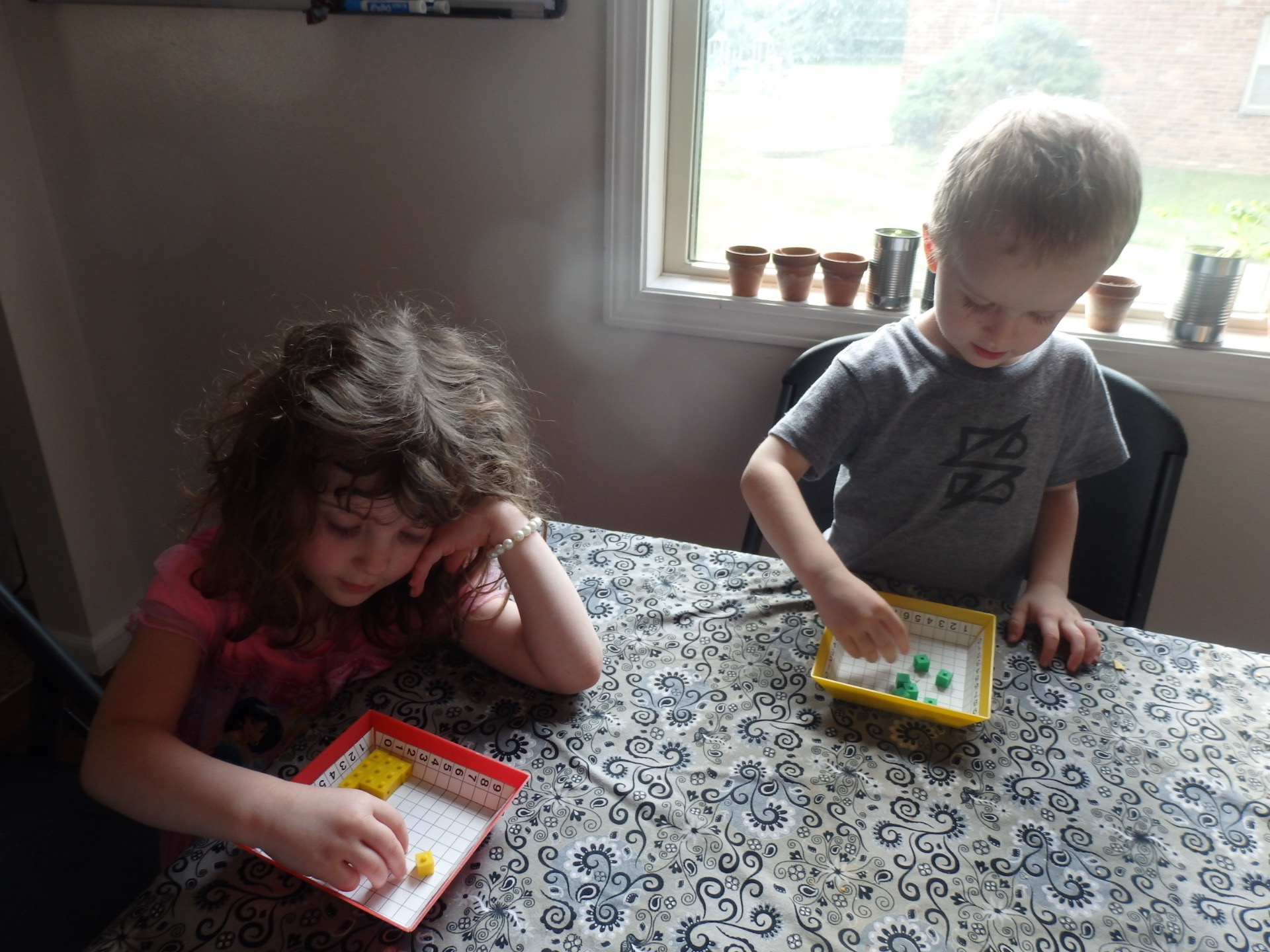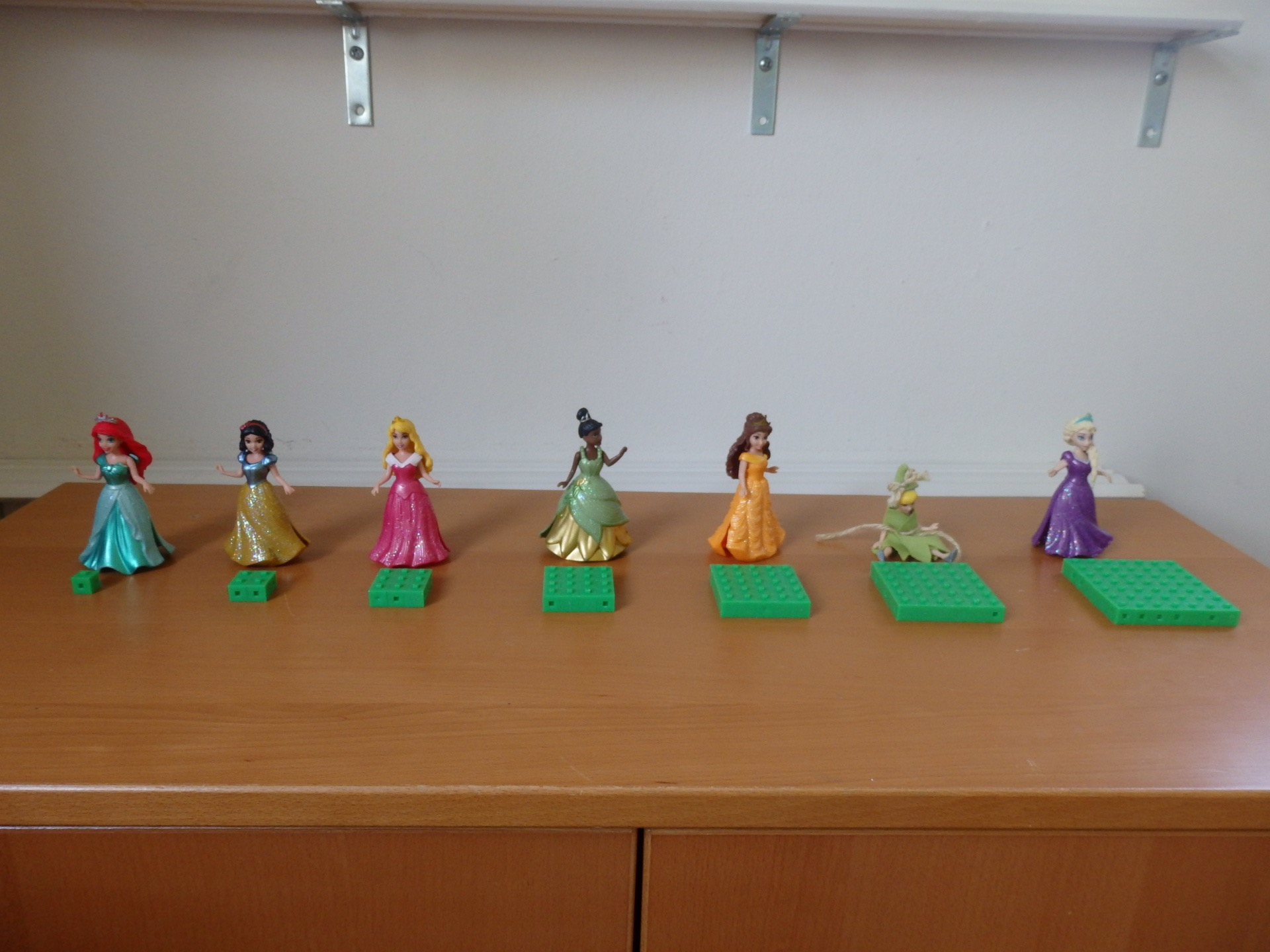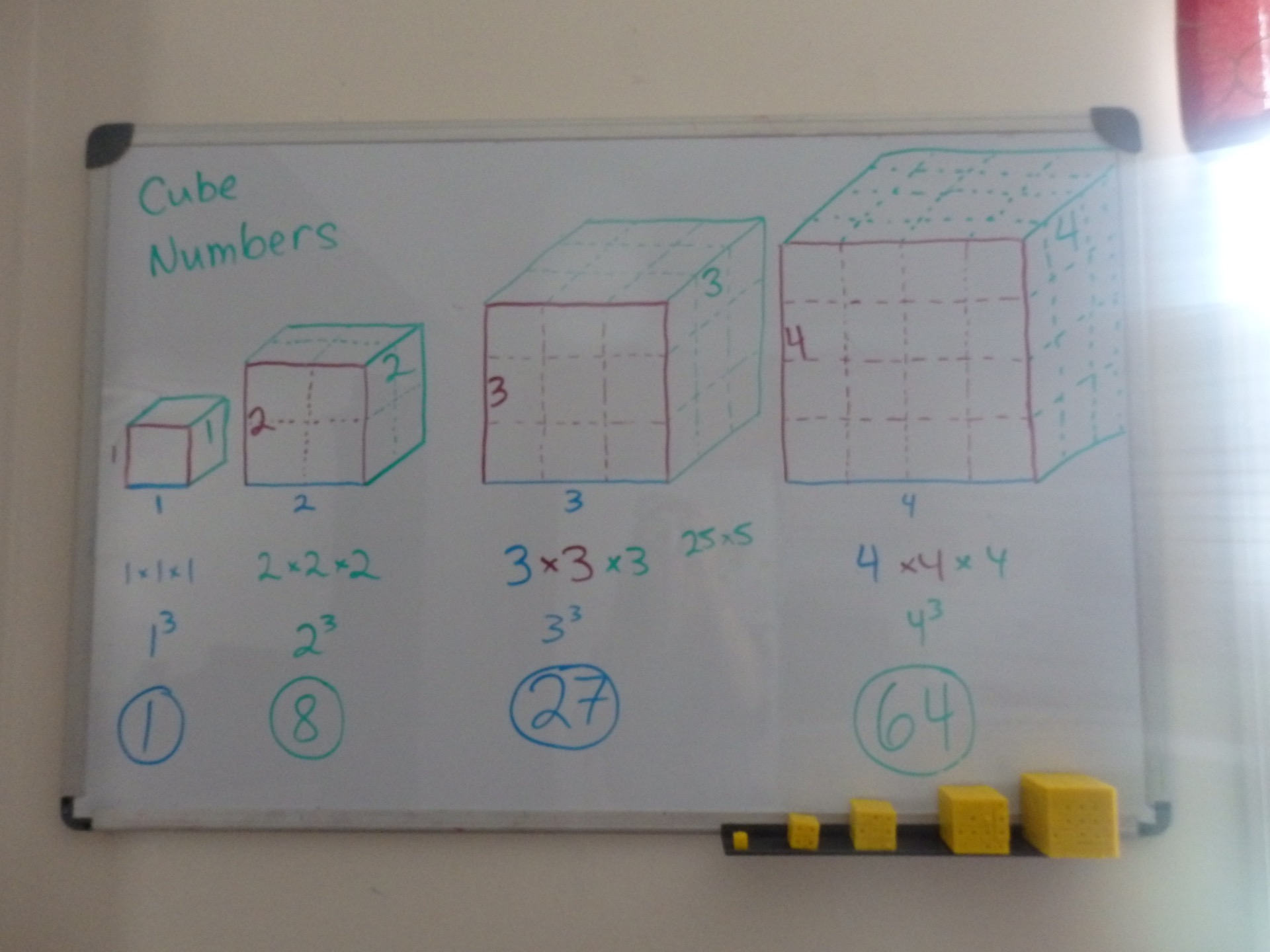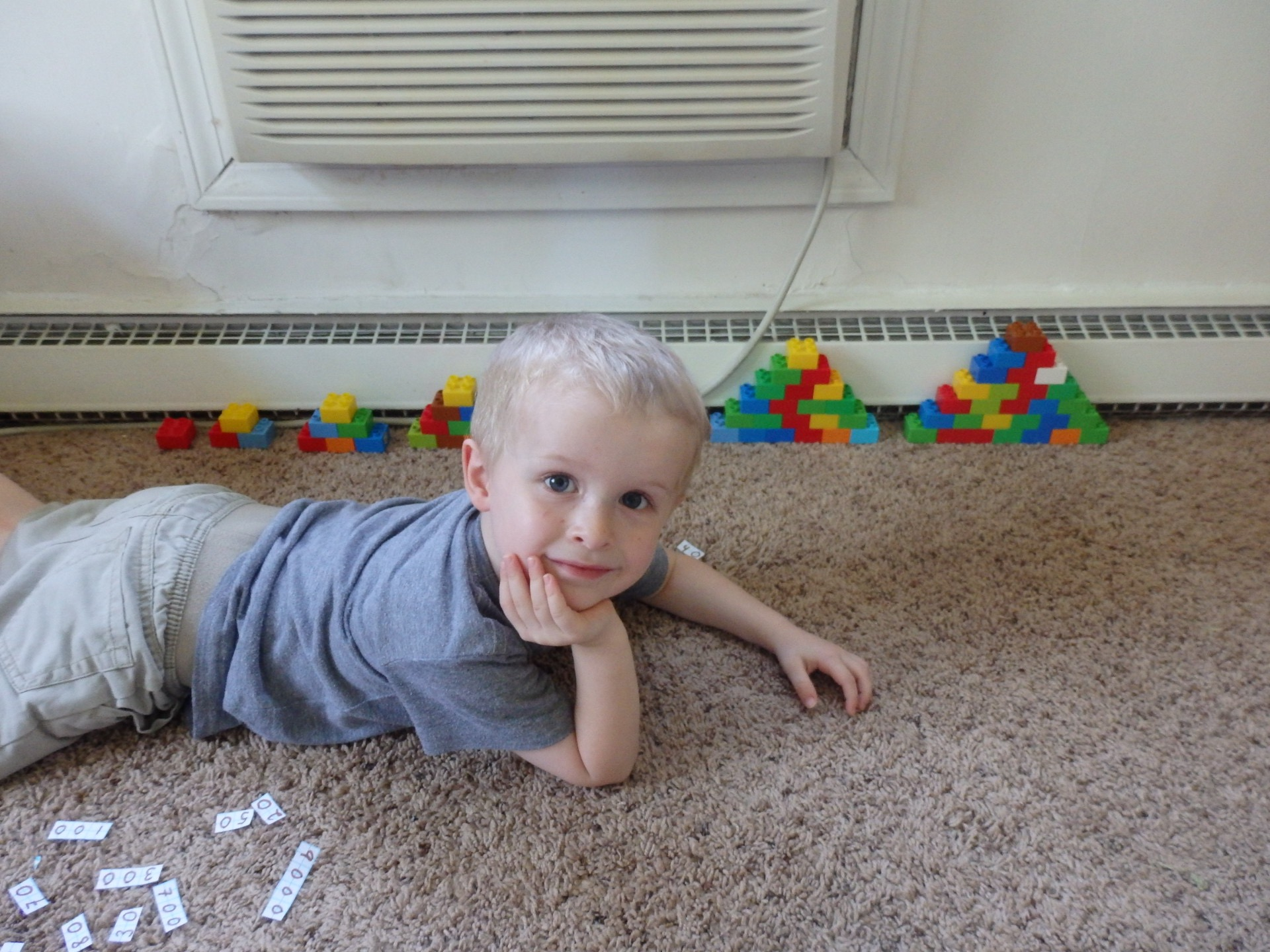Did you know that some numbers have shapes?

Some are square, like 1, 4, 9, and 16.

You probably didn’t know that fairies only like square pies. So it’s important to know which numbers are square, because if you make a rectangular pie for a fairy she won’t eat it. Sparrow men (boy fairies) will eat other shapes, but fairies will not.

Heidi and Peter are trying to figure out if their number is square.Some numbers are cubic, like 1, 8, 27, 64, and 125. Fairies only like cubic presents.I recently learned that some are triangular. 1, 3, 6, 10, 15, 21, and so on.The first pyramid is made of 1, the second is made of 1+2=3, the third is 1+2+3=6, the fourth is 1+2+3+4=10, etc. Peter likes stuff like that, so we had fun.